•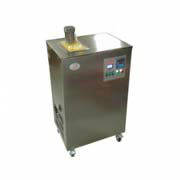标准恒温油槽 `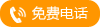` `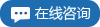`
•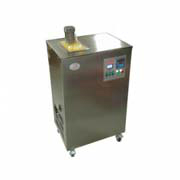标准恒温水槽 `` ``
•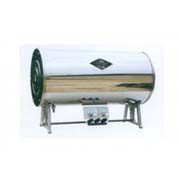热电偶检定炉 `` ``
•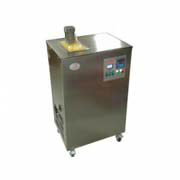XYS-II型制冷恒 `` ``
•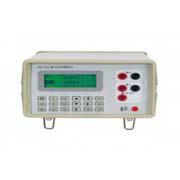热工信号校验仪 `` ``
•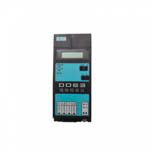D063现场校验仪 `` ``
•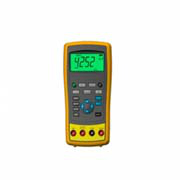热电偶校验仪（ `` ``
•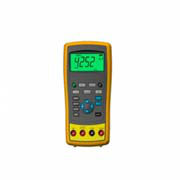热电阻校验仪（ `` ``
•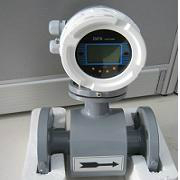高精度电磁流量 `` ``
•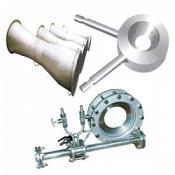流量测量节流装 `` ``
•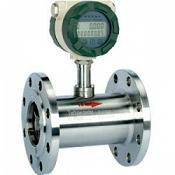液体涡轮流量计 `` ``
•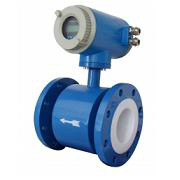一体式电磁流量 `` ``
•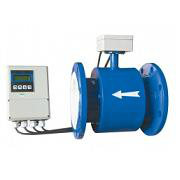污水流量计(分体 `` ``
•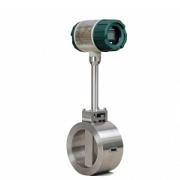智能一体式涡街 `` ``
•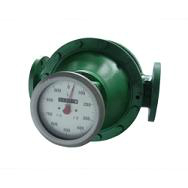椭圆齿轮流量计 `` ``
•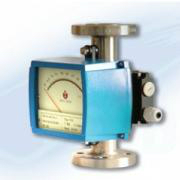金属管转子流量 `` ``
•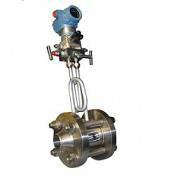一体化孔板流量 `` ``
•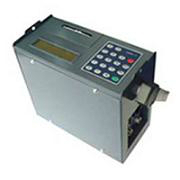便携式超声波流 `` ``
•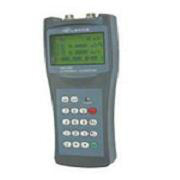手持式超声波流 `` ``
•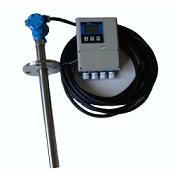插入式电磁流量 `` ``